[Introduction]  [Signal arithmetic]  [Signals and noise]   [Smoothing]   [Differentiation]  [Peak Sharpening]  [Harmonic analysis]   [Fourier convolution]  [Fourier deconvolution]  [Fourier filter]  [Wavelets]   [Peak area measurement]  [Linear Least Squares]  [Multicomponent Spectroscopy]  [Iterative Curve Fitting]  [Hyperlinear quantitative absorption spectrophotometry] [Appendix and Case Studies]  [Peak Finding and Measurement]  [iPeak]   [iSignal]  [Peak Fitters]   [iFilter]  [iPower]  [List of downloadable software]  [Interactive tools]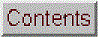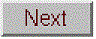## iSignal: Interactive smoothing, differentiation, and signal analysis

### [Smoothing]  [Differentiation]  [Peak Sharpening]  [Peak de-tailing]  [Convolution/Deconvolution]  [Background subtraction]  [Peak measurement]  [Peak Fitting]  [Polynomial fitting]  [Frequency Spectrum]  [Examples]  [Playing sounds] [Matlab/Octave command-line function]  [Have a question? Email me]

Last few versions: Version 8.3, May 2020 add constant denominator addition to deconvolution options, to reduce ringing and noise (the "5" and "6" keys change the added constant). Version 8.2, April 2020. Interactive convolution and deconvolution, "Shift V" key to select from menu; "3" and "4" keys change the width by 10% and "Shift-3" and "Shift-4" keys change by 1%. Version 7, June 2019. Symmetrize exponentially broadened peaks peaks by weighted first derivative addition ("Shift Y" key to enter weighting factor; "1" and "2" keys change weighting factor by 10% and "Shift-1" and "Shift-2" keys change by 1%).  Version 6, December 2017 adds a segmented smooth (Shift-Q) and a function to compare signals before and after processing (Shift-B). Version 5.95,  May, 2017, adds ^ (Shift-6) Raises the signal to the specified power. Version 5.9 added a peak finding function based on the autopeaks function, activated by the J or Shift-J keys.. (The demo script "demoisignal.m" is a self-running demonstration of several features of the program and will test for proper installation; the title of each figure describes what is happening).

Its basic operation is similar to iPeak
and ipf.m. The syntax is:

pY=isignal(Data);

or

[pY,PowerSpectrum,maxy,miny,area,stdev] = isignal(datamatrix,xcenter,xrange,sm,sw,em,dm,rm,K1,K2,sr,sz,sf,mw,spm)

"Data" may be a 2-column matrix with the independent variable (x-values) in the first column and dependent variable (y values) in the second column, or separate x and y vectors, or a single y-vector (in which case the data points are plotted against their index numbers on the x axis). Only the first argument (Data) is required; all the others are optional. Returns the processed dependent axis ('pY') vector (and, in the Spectrum Mode, the frequency spectrum matrix, 'Spectrum') as the output arguments. Plots the data in the figure window, the lower half of the window show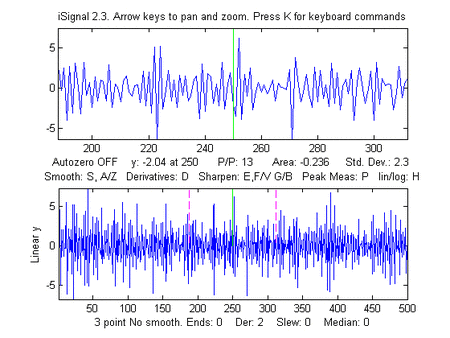ing the entire signal, and the upper half showing a selected portion controlled by the pan and zoom keys (the four cursor arrow keys), with the initial pan and zoom settings optionally controlled by input arguments 'xcenter' and 'xrange', respectively. Other keystrokes allow you to control the smooth type, width, and ends treatment, the derivative order (0th through 5th), and peak sharpening. (The initial values of all these parameters can be passed to the function via the optional input arguments SmoothMode, SmoothWidth, ends, DerivativeMode, Sharpen, Sharp1, Sharp2, SlewRate, and MedianWidth. See the examples below). Press K to see all the keyboard commands. Note: Make sure you don't click on the "Show Plot Tools" button in the toolbar above the figure; that will disable normal program functioning. If you do; close the Figure window and start again.

Smoothing
The S key (or input argument "SmoothMode") cycles through five smoothing modes:
If SmoothMode=0, the signal is not smoothed.
If SmoothMode=1, rectangular (sliding-average or boxcar)
If SmoothMode=2, triangular (2 passes of sliding-average)
If SmoothMode=3, pseudo-Gaussian (3 passes of sliding-average)
If SmoothMode=4, Savitzky-Golay smooth (thanks to Diederick).

The A and Z keys (or optional input argument SmoothWidth) control the SmoothWidth, w. Example shown in the figure on the right.

To specify a segmented smooth, press Shift-Q. You can specify the smooth width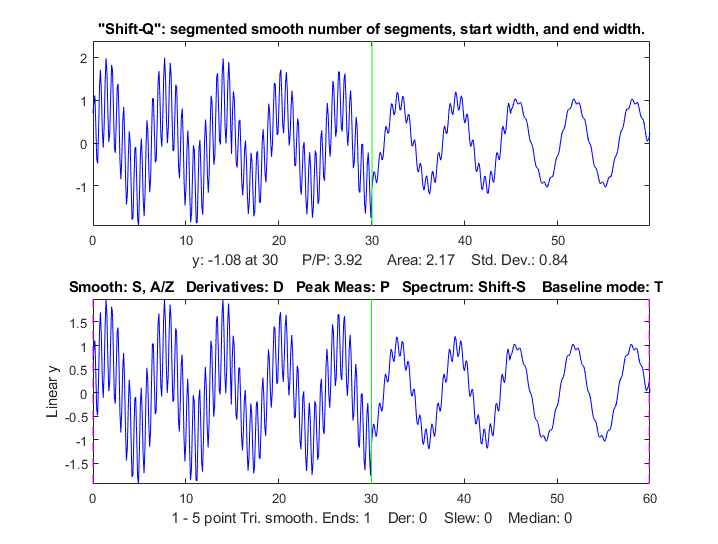vector in two ways: at the prompt you can (a) enter the number of segments (then you'll be prompted to enter the smooth widths in the first and last segments, and the computer will calculate integer values of smooth widths that are evenly divided between the specified first and last values, or (b) type in the smooth width vector directly including the square brackets, e.g. [1 3 3 9]. In either case, subsequently adjusting the smooth width with the A and Z keys will vary all the segments by the same percentage factor. (To return to an ordinary single segment smooth, enter 1 as the number of segments). Picture on right.

The X key toggles "ends" between 0 and 1. This determines how the "ends" of the signal (the first w/2 points and the last w/2 points) are handled when smoothing:
If ends=0, the ends are zero.
If ends=1, the ends are smoothed with progressively smaller smooths the closer to the end. Generally, ends=1 is best, except in some cases using the derivative mode when ends=0 result in better vertical centering of the signal.

Note: when you are smoothing peaks, you can easily measure the effect of smoothing on peak height and width by turning on peak measure mode (press P) and then press S to cycle through the smooth modes.

T
here are two special functions for removing or reducing sharp spikes in signals: the M key, which implements a median filter (it asks you to enter the spike width, e.g. 1,2, 3... points) and the ~ key, which limits the maximum rate of change.
Reference: http://terpconnect.umd.edu/~toh/spectrum/Smoothing.html

Differentiation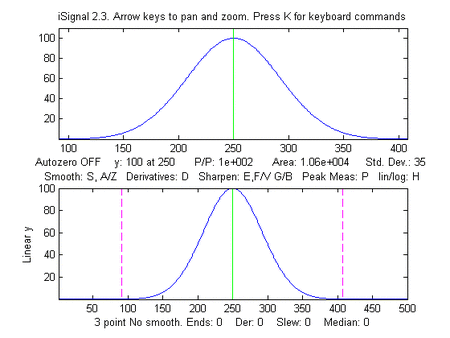The
D / Shift-D keys (or optional input argument "DerivativeMode") increase/decrease the derivative order. The default is 0. Careful optimization of smoothing of derivatives is critical for acceptable signal-to-noise ratio. Example shown in the figure on the right. In SmoothModes 1 through 3, the derivatives are computed with respect to the independent variable (x-values), corrected for non-uniform x axis intervals. In SmoothMode 4 (Savitzky-Golay) the derivatives are computed by the Savitzky-Golay algorithm.
Reference: http://terpconnect.umd.edu/~toh/spectrum/Differentiation.html

Peak sharpening (resolution enhancement)
The E key (or optional input argument "Sharpen") turns off and on peak sharpening (resolution enhancement) by the even-derivative technique. The sharpening strength is controlled by the F and V keys (or optional input argument "Sharp1") and B and G keys (or optional argument "Sharp2"). The optimum values depend on the peak shape and width. For peaks of Gaussian shape, a reasonable value for Sharp1 is PeakWidth2/25 and for Sharp2 is PeakWidth4/800 (or PeakWidth2/6 and PeakWidth4/700 for Lorentzian peaks), where PeakWidth is the full-width at half maximum of the peaks expressed in number of data points.  However, you don't need to do the math yourself; iSignal can calculate sharpening and smoothing settings for Gaussian and for Lorentzian peak shapes using the Y and U keys, respectively. Just isolate a single typical peak in the upper window using the pan and zoom keys, then press Y for Gaussian or U for Lorentzian peaks.  (The optimum settings depends on the width of the peak, so if your signal has peaks of widely different widths, o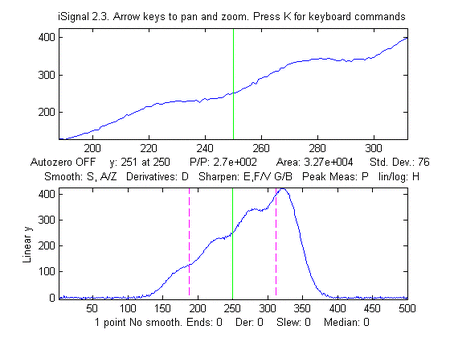ne setting will not be optimum for all the peaks). You can fine-tune the sharpening with the F/V and G/B keys and the smoothing with the A/Z keys.

You can expect a decrease in peak width (and corresponding increase in peak height) of about 20% - 50%, depending on the shape of the peak (the peak area is largely unaffected by sharpening).  Excessive sharpening leads to baseline artifacts and increased noise. iSignal allows you to experimentally determine the values of these parameters that give the best trade-off between sharpening, noise, and baseline artifacts, for your purposes.
You can easily measure the effect of sharpening quantitatively by turning on peak measure mode (press P) and then press E to toggle the sharpen mode off and on. Note: only the Savitzky-Golay smooth mode is used for peak sharpening. Example shown in the figure on the right.

If your s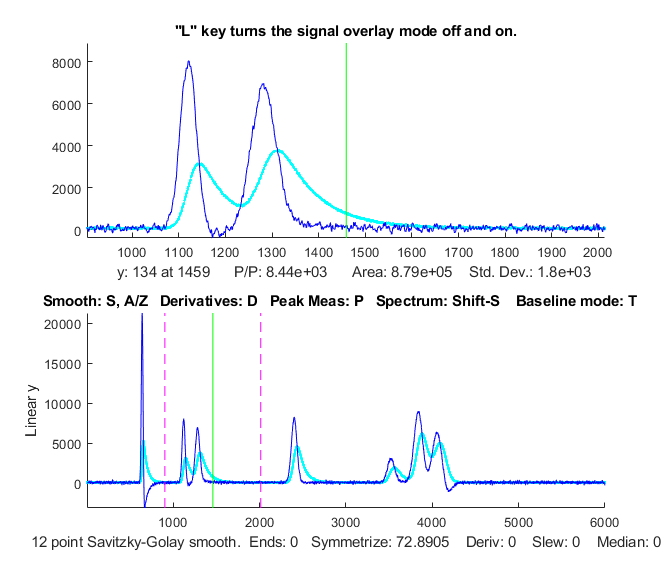ignal has peaks that are exponentially broadened, you can remove that broadening, and increase the symmetry of the peaks, by the weighted first-derivative addition technique. Press Shift-Y and enter an estimated weighting factor (e.g., start with the width of the peak), then press the "1" and "2" keys to change weighting factor by 10% and the "Shift-1" and "Shift-2" keys to change by 1%. Increase the factor until the baseline after the peak goes negative, then increase it slightly so that it is as low as possible but not negative. Adjust the smoothing to control the noise (the Savitsky-Golay smooth is ideal here). The result is narrower, taller peaks, but with the peak areas. It has the same effect as deconvoluting the exponential function from the broadened signal, but it is faster and simpler. It the peaks tail to the left rather than the right, use a negative weighting factor. To cancel the effect, press Shift-Y and set the time constant to zero. Run the script iSignalSymmTest.m for a example signal with two overlapping exponentially broadened Gaussians. The graphic on the left shows a real-data example, after which the L key was pressed to overlay and compare the de-tailed peaks (in blue) with the original signal (in cyan). If desired, and if the signal-to-noise ratio is not too poor, the de-tailed peaks can be further sharpened using the even-derivative technique (E key, previous section).

Interactive convolution and deconvolution

In iSignal 8.3 you can press Shift-V to display the menu of Fourier convolution and deconvolution operations that allow you to convolute a Gaussian or exponential function with the signal, or to deconvolute a Gaussian or exponential function from the signal. It will ask you for the width or the time constant (in x units).

1. Convolution
2. Deconvolution
Select mode 1 or 2:  2

Shape of convolution/deconvolution function:
1. Gaussian
2. Lorentzian
3. Exponential
Select shape 1, 2, or 3:  2

Enter the width in x units: 2.1

Enter Denominator Addition, zero to disable: 0.1

Then you enter the time constant (in x units) and press Enter. Then use the 3 and 4 keys to adjust the width of the deconvolution function by 10% (or Shift-3 and Shift-4 to adjust by 1%). You may need to adjust the smoothing if the signal is too noise, but too much smoothing will broaden the peaks. This version of iSignal includes an additional way to reduce ringing and noise in the deconvoluted signal, by adding a constant to the denominator (reference 86) and adjusting it with the 5 and 6 keys to decrease or increase the constant by 10% (or Shift-5 and Shift-6 to adjust by 1%).

The example on the right show the self-deconvolution of a Lorentzian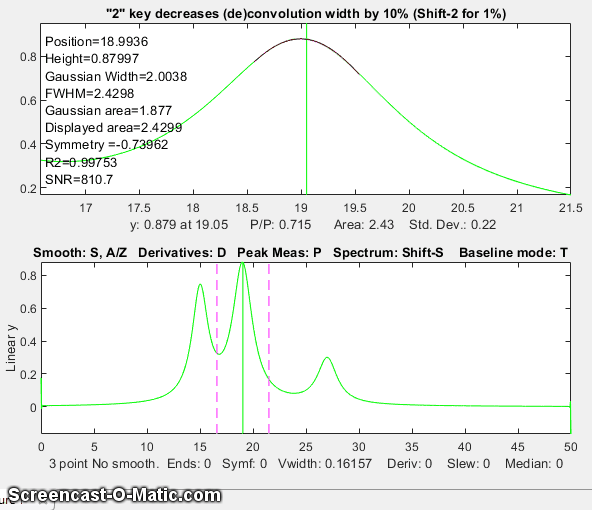from a group of three overlapping Lorentzians in a effort to narrow the peaks. The screen shows the signal zoomed to the middle peak (top panel). The entire signal is shown in the bottom panel. The animation shows what happens when you increase the width of the deconvolution Lorentzian ("Vwidth", displayed at the bottom of the lower panel) from 0.16 to 2.5 and then back drown to around 2. (I do that with the 3 and 4 keys). Watch the close-up of the center peak in the upper panel, which also measures the peak position, height, with and area continuously. The height goes up and the width goes down. How far can you go with this?  In many experimental domains, the signal is expected to be positive, except for instances where random noise on the baseline may make the signal occasionally slightly negative. If you increase the deconvolution width too far, the peaks will develop negative dips on their sides and will become excessively noisy. That sets an upper limit to the deconvolution with that can be employed, but that has to be determined experimentally on a case-by-case basis, guided by the needs of the experimenter. For a real-data example, see Deconvolution.html#Data658. Note: in cases where the peak widths within a group of peaks are substantially different, you can use segmented deconvolution.

Signal measurement
The cursor keys control the position of the green cursor (left and right arrow keys) and the distance between the dotted red cursors (up and down arrow keys) that mark the selected range displayed in the upper graph window. The label under the top graph window shows the value of the signal (y) at the green cursor, the peak-to-peak (min and max) signal range, the area under the signal, and the standard deviation within the selected range (the dotted cursors). Pressing the Q key prints out a table of the signal information in the command window.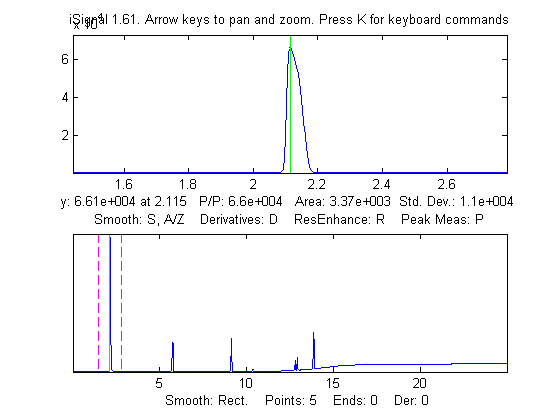Signal is measured by placing the green center cursor on top of the peak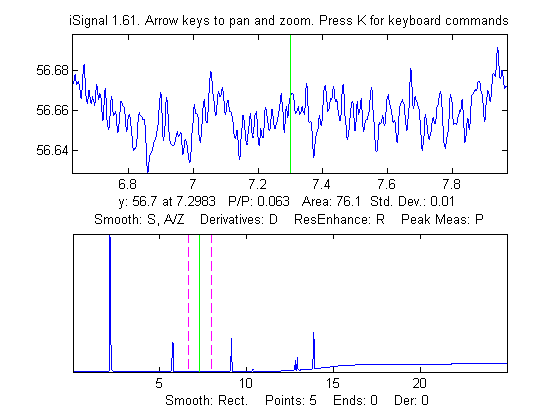Noise is the standard deviation measured on a flat portion of the baseline

Signal-to-noise ratio (SNR) measurement of a signal with very high SNR.  Left: The peak height of the largest signal peak is measured by placing the green center cursor on the largest peak; peak-to-peak signal=66,000. Right: The noise is measured on a flat portion of the baseline: standard deviation of noise=0.01, therefore the SNR=66,000/.01 = 6,600,000

If the optional output arguments maxy, miny, area, stdev are specified, isignal returns the maximum value of y, the minimum value of y, the total area under the curve, and the standard deviation of y, in the selected range displayed in the upper panel.

Frequency Spectrum mode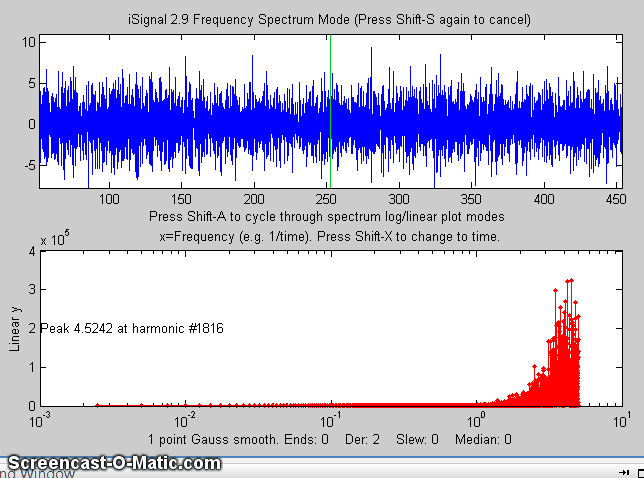The Frequency Spectrum mode, toggled on and off by the Shift-S key, computes the Fourier frequency spectrum of the segment of the signal displayed in the upper window and displays it in the lower window (temporarily replacing the full-signal display). Use the pan and zoom keys to adjust the region of the signal to be viewed. Press Shift-A to cycle through four plot modes (linear, semilog X, semilog Y, or log-log) and press Shift-X to toggle between a frequency on the x axis and time on the x-axis.  All signal processing functions remain active in the frequency spectrum mode  (smooth, derivative, etc) so you can observe the effect of these functions on the frequency spectrum of the signal, as in the animated figure on the right. Press Shift-T to transfer the frequency spectrum to the signal in the upper panel, so you can pan and zoom and do other processing and measurements on the frequency spectrum. Press Shift-S again to return to the normal mode. Spectrum mode is a visible mode, indicated by the label at the top of the figure. To start off in the spectrum mode, set the 13th input argument, SpectrumMode, to 1. To save the spectrum as a new variable, call isignal with the output arguments [pY,Spectrum]:
>> x=0:.1:60; y=sin(x)+sin(10.*x);
>> [pY,Spectrum]=isignal([x;y],30,30,4,3,1,0,0,1,0,0,0,1);
>> plot(Spectrum(:,1),Spectrum(:,2)) or plotit(Spectrum) or isignal(Spectrum); or ipf(Spectrum); or ipeak(Spectrum); or whatever.
Shift-Z toggles on and off peak detection and labeling on the frequency/time spectrum; peaks are labeled with their frequencies. You can adjust the peak detection parameters in lines 2192-2195 in version 5; see http://terpconnect.umd.edu/~toh/spectrum/PeakFindingandMeasurement.htm. The Shift-W command displays the 3D waterfall spectrum, by dividing up the signal into segments and computing the power spectrum of each segment. This is mostly a novelty, but it may be useful for signals whose frequency spectrum varies over the duration of the signal. You are asked to choose the number of segments into which to divide the signal (that is, the number of spectra) and the type of 3D display (mesh, contour, surface, etc).
Background subtraction
There are two ways to subtract the background from the signal: automatic and manual. To select an automatic baseline correction mode, press the T key repeatedly; it cycles thorough four modes: No baseline correction, linear baseline subtraction, quadratic baseline subtraction, flat baseline correction, then back to no baseline correction.  When baseline mode is linear, a straight-line baseline connecting the two ends of the signal segment in the upper panel will be automatically subtracted. When baseline mode is quadratic, a parabolic baseline connecting the two ends of the signal segment in the upper panel will be automatically subtracted. The baseline is calculated by computing a linear (or quadratic) least-squares fit to the signal in the first 1/10th of the points and the last 1/10th of the points. Try to adjust the pan and zoom to include some of the baseline at the beginning and end of the segment in the upper window, allowing the automatic baseline subtract gets a good reading of the baseline. The flat baseline mode is used only for peak fitting. The calculation of the signal amplitude, peak-to-peak signal, and peak area are all recalculated based on the baseline-subtracted signal in the upper window. If you are measuring peaks superimposed on a background, the use of the autozero mode will have a big effect on the measured peak height, width, and area, but very little effect on the peak x-axis position, as demonstrated by these two figures.

In addition to the four autozero baseline subtraction modes for peak measurement, a manually estimated piecewise linear baseline can be subtracted from the entire signal in one operation. The Backspace key starts background correction operation. In the command window, type in the number of background points to click and press the Enter key. The cursor changes to crosshairs; click along the presumed background in the figure window, starting to the left of the x axis and placing the last click to the right of the x axis. When the last point is clicked, the linearly interpolated baseline between those points is subtracted from the signal. To restore the original background (i.e. to start over), press the '\' key (just below the backspace key).

Peak and valley measurement
The P key toggles off and on the "peak parabola" mode, which attempts to measure the one peak (or valley) that is centered in the upper window under the green cursor by superimposing a least-squares best-fit parabola, in red, on the center portion of the signal displayed in the upper window. (Zoom in so that the red overlays just the top of the peak or the bottom of the valley as closely as possible). The peak position, height, and width are measured by least-squares curve fitting of a Gaussian to the central peak over the segment that is colored red in the upper panel. (Change the pan and zoom to modify that region; the readings will change as the segment measured is changed). The "RSquared" value is the coefficient of determination; the closer to 1.000 the better. The peak parameters will most accurate if the peaks are Gaussian. Other peak shapes, or very noisy peaks of any shape, will give only approximate results. However, the position and height, and area values are pretty good for any peak shape as long as the "RSquared" value is at least 0.99. The "SNR" is the signal-to-noise-ratio of the peak under the green cursor; it's the ratio of the peak height to the standard deviation of the residuals between the data and the best-fit line in red. Example shown in the figure on the right. If the peaks are superimposed on a non-zero background, subtract the background before measuring peaks, either by using the autozero mode (T key) or the multi-point background subtraction (backspace key). Press the R key to print out the peak parameters in the command window.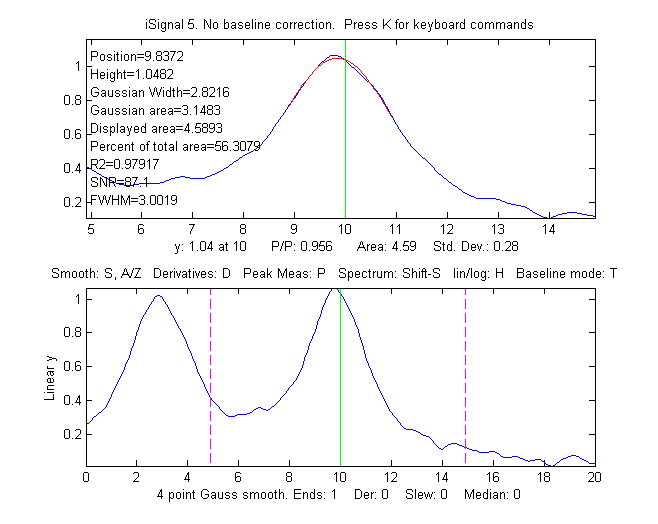Peak width is actually measured two ways: the "Gaussian Width" is the width measured by Gaussian curve fitting (over the region colored in red in the upper panel) and is strictly accurate only for Gaussian peaks. Version 5.8 (shown below on the left) adds direct measurement of the full width at half maximum ('FWHM') of the central peak in the upper panel (the peak marked by the green vertical line); this works for peaks of any shape, but it is computed only for the central peak and only if the half-maximum points fall within the zoom region displayed in the upper panel (otherwise it will return NaN). It will not be highly accurate for very noisy peaks. The Gaussian width will be more accurate for noisy or sparsely sampled peaks, but only if the peaks are at least approximately Gaussian. In the example on the right, the peaks are Lorentzian, with a true with of 3.0, plus added noise. In this case the measured FWHM (3.002) is more accurate that the Gaussian width (2.82), especially if a little smoothing is used to reduce the noise.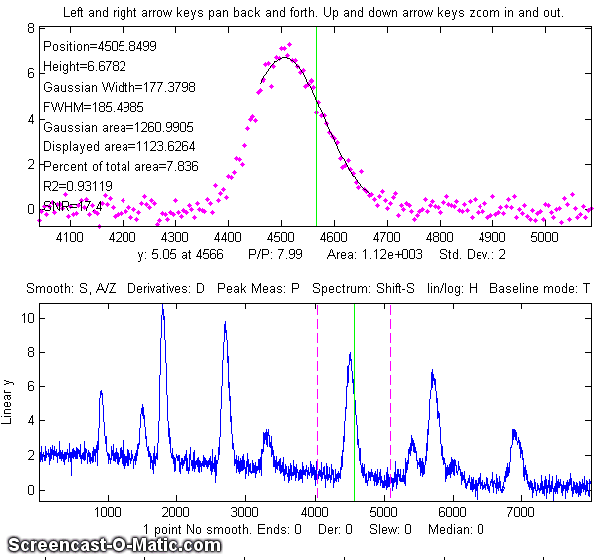Peak area is also measured two ways: the "Gaussian area" and the "Total area". The "Gaussian area"  is the area under the Gaussian that is best fit to the center portion of the signal displayed in the upper window, marked in red.  The "Total area" is the area by the trapezoidal method over the entire selected segment displayed in the upper window. (The percent of total area is also calculated). If the portion of the signal displayed in the upper window is a pure Gaussian with no noise and a zero baseline, then the two measures should agree almost exactly.  If the peak is not Gaussian in shape, then the total area is likely to be more accurate, as long as the peak is well separated from other peaks. If the peaks are overlapped, but have a known shape, then peak fitting (Shift-F) will give more accurate peak areas. In the example above on the right, the Lorentzian  peak at x=10 has a true area of 4.488, so in this case the total area (4.59) is more accurate than the Gaussian area (3.14), but it is too high because of overlap with the peak at x=3. Curve fitting both Lorentzians peaks together would yield the most accurate areas. If the signal is panned slightly left and right, using the left and right cursor keys or the "[" and "]" keys, the peak parameters displayed will change slightly due to the noise in the data - the more noise, the greater the change, as in the example on the left. If the peak is asymmetrical, as in this example, the peak widths displayed on one side will be greater that the other side.

In version 5.9 there is an automatic peak finder that is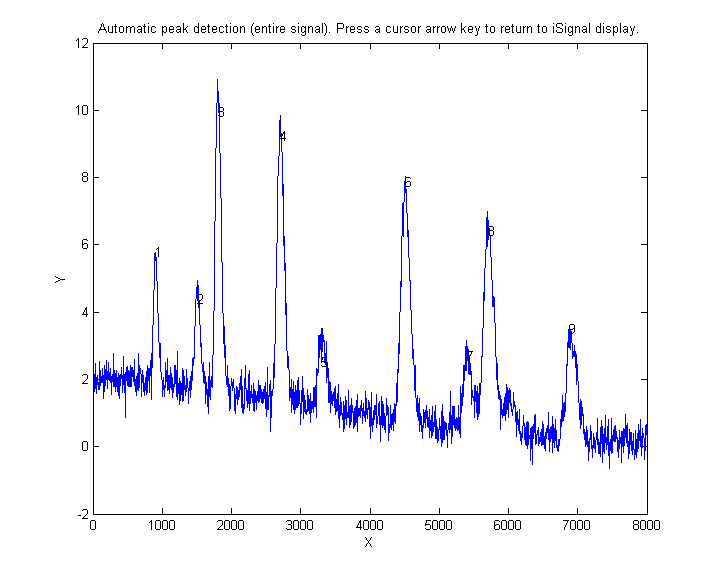based on the autopeaks.m function (activated by the J key); it asks for the peak density (roughly the number of peaks that fit into the signal record), then detects, measures, and displays the peak position, height, and area of all the peaks it detects in the processed signal currently displayed in the lower panel, plots and number the peaks as shown on the right and also plots each peak separately in Figure(2) with peak, tangent, and valley points marked. (The peak density number controls the peaks sensitivity - larger numbers cause the routine to detect larger numbers of narrower peaks, and smaller numbers ignore the fine structure and looks for broader peaks). It also prints out the peak detection parameters that it calculates for use by any of the findpeaks... functions. To return to the usual iSignal display, press any cursor arrow key. (Shift-J does the same thing for the segment displayed in the upper window).

Peak fitting (NEW PROCEDURE in version 5 and later)
iSignal has an iterative curve fitting method performed by peakfit.m. This is the most accurate method for the measurements of the areas of highly overlapped peaks. First, center the signal you wish to fit using the pan and zoom keys (cursor arrow keys), select the baseline mode by pressing the 'T' key to cycle through the 4 modes. Press the Shift-F key, then type the desired peak shape by number from the menu displayed in the Command window (shown below), enter the number of peaks, enter the number of repeat trial fits (usually 1-10), and finally click the mouse pointer on the top graph where you think the peaks might be. A graph of the fit is displayed in Figure window 2 and a table of results is printed out in the command window. Version 5 of iSignal can fit many different combinations of peak shapes and constraints:

Gaussians: y=exp(-((x-pos)./(0.6005615.*width)) .^2)
Gaussians with independent positions and widths.....................1 (default)
Gaussians with the same widths......................................6
Gaussians with preset fixed widths.................................11
Fixed-position Gaussians...........................................16
Asymmetrical Gaussians with unequal half-widths on both sides......14
Lorentzians: y=ones(size(x))./(1+((x-pos)./(0.5.*width)).^2)
Lorentzians with independent positions and widths...................2
Equal-width Lorentzians.............................................7
Fixed-width Lorentzian.............................................12
Fixed-position Lorentzian..........................................17
Gaussian/Lorentzian blend (equal blends).............................13
Fixed-width Gaussian/Lorentzian blend..............................35
Gaussian/Lorentzian blend with independent blends).................33
Voigt profile with equal alphas).....................................20
Fixed-width Voigt profile with equal alphas........................34
Voigt profile with independent alphas..............................30
Logistic: n=exp(-((x-pos)/(.477.*wid)).^2); y=(2.*n)./(1+n)...........3
Pearson: y=ones(size(x))./(1+((x-pos)./((0.5.^(2/m)).*wid)).^2).^m....4
Fixed-width Pearson................................................37
Pearson with independent shape factors, m..........................32
Breit-Wigner-Fano....................................................15
Exponential pulse: y=(x-tau2)./tau1.*exp(1-(x-tau2)./tau1)............9
Alpha function: y=(x-spoint)./pos.*exp(1-(x-spoint)./pos);...........19
Up Sigmoid (logistic function): y=.5+.5*erf((x-tau1)/sqrt(2*tau2))...10
Down Sigmoid y=.5-.5*erf((x-tau1)/sqrt(2*tau2))......................23
Triangular...........................................................21

Note: if you have a peak that is an exponentially-broadened Gaussian or Lorentzian, you can measure both the "after-broadening" height, position, and width using the P key function, and the "before-broadening" height, position, and approximate width by fitting the peak to an exponentially-broadened Gaussian or Lorentzian model (shapes 5, 8,36, 31, or 18) using the Shift-F key function. The peak areas will be the same; broadening does not effect the total peak area.

Polynomial fitting.

Shift-o fits a simple polynomial (linear, quadratic, cubic, etc) to the upper panel segment and displays the coefficients (in descending powers) and the correlation coefficient R2
Saving the results
To save the processed signal to the disc as an x,y matrix in mat format, press the 'o' key, then type in the desired file name into the "File name" field, then press Enter or click Save. To load into the workspace, type "load" followed by the file name you typed. The processed signal will be saved in a matrix called "Output"; to plot the processed data, type "plot(Output(:,1),Output(:,2))".

Other keystroke controls
The Shift-G key toggles on and off a temporary grid on the upper and lower panel plots. The L key toggles off and on the Overlay mode, which shows the original signal as a dotted line overlaid on the current processed signal, for the purposes of comparison. Shift-B opens Figure window 2 and plots the original signal in the upper panel and the processed signal in the lower panel (graphic). The Tab key restores the original signal and cursor settings. The ";" key sets the selected region to zero (to eliminate artifacts and spikes). The "-" (minus sign) key is used to negate the signal (flip + for -).  Press H to toggle display of semilog y plot in the lower window, which is useful for signals with very wide dynamic range, as in the example in the figures below (zero and negative points are ignored in the log plot). Press '+' key to take the absolute value of the entire signal (and follow this by a smooth to create an amplitude modulation "detector"). In version 5.7, Shift-L replaces the signal with the processed version of itself, for the purpose of applying more passes of different widths of smoothing or higher orders of differentiation. In version 5.95, the ^ (Shift-6) raises the signal to the specified power. To reverse this, simply raise to the reciprocal power. See Power transform method of peak sharpening.
 Linear y-axis mode Log y mode (H key)

The C key condenses the signal by the specified factor n, replacing each group of n points with their average (n must be an integer, such as 2,3, 4, etc). The I key replaces the signal with a linearly interpolated version containing m data points. This can be used either to increase or decrease the x-axis interval of the signal or to convert unevenly spaced values to evenly spaced values. After pressing C or I, you must type in the value of n or m respectively.

You can press Shift-C, then click on the graph to print out the x,y coordinates of that point.  This works on both the upper and lower panels, and on the frequency spectrum as well.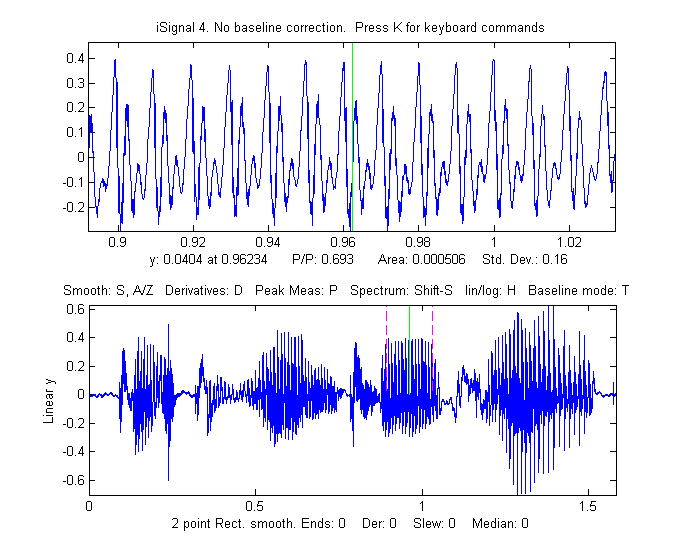Playing data as audio.
Press Spacebar or Shift-P to play the segment of the signal displayed in the upper window as audio through the computer's sound output. Press Shift-R to set the sampling rate - the larger the number the shorter and higher-pitched will be the sound. The default rate is 44000Hz. Sounds or music files in WAV format can be loaded into Matlab using the built-in "wavread" function. The example on the right shows a 1.5825 sec duration audio recording of the phrase "Testing, one, two, three" recorded at 44000 Hz, saved in WAV format (link), loaded into iSignal and zoomed in on the "oo" sound in the word "two". Press Spacebar to play the selected sound; press Shift-S to display the frequency spectrum of the selected region, and press Shift-Z to label the peaks in the frequency spectrum with their frequencies (graphic).

>> t=0:1/44001:1.5825;
>> isignal(t,v(:,2));

Press Shift-R and type 44000 to set the sampling rate. This recorded sound example allows you to experiment with the effect of smoothing, differentiation, and interpolation on the sound of recorded speech. Interestingly, different degrees of smoothing and differentiation will change the timbre of the voice but has little effect on the intelligibility. This is because the sequence of frequency components in the signal is not shifted in pitch or in time but merely changed in amplitude by smoothing and differentiation. Even computing the absolute value (+ key), which effectively doubles the fundamental frequency, does not make the sound unintelligible.

Shift-Ctrl-F transfers the current signal to Interactive Peak Fitter (ipf.m) and Shift-Ctrl-P  transfers the current signal to Interactive Peak Detector (iPeak.m), if those functions are installed in your Matlab path.

Press K to see all the keyboard commands.

EXAMPLE 1: Single input argument; data in a two columns of a matrix [x;y] or in a single y vector
>> isignal(y);
>> isignal([x;y]);

EXAMPLE 2: Two input arguments. Data in separate x and y vectors.
>> isignal(x,y);

EXAMPLE 3: Three or four input arguments. The last two arguments specify the initial values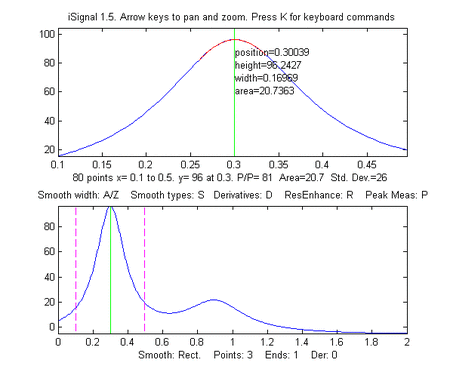of pan (xcenter) and zoom (xrange) in the last two input arguments. Using data in the ZIP file:

>> isignal(DataMatrix,180,40); or
>> isignal(x,y,180,40);

EXAMPLE 4: As above, but additionally specifies initial values of SmoothMode, SmoothWidth, ends, and DerivativeMode in the last four input arguments.
>> isignal(DataMatrix,180,40,2,9,0,1);

EXAMPLE 5: As above, but additionally specifies initial values of the peak sharpening parameters Sharpen, Sharp1, and Sharp2 in the last three input arguments.  Press the E key to toggle sharpening on and off for comparison.
>> isignal(DataMatrix,180,40,4,19,0,0,1,51,6000);

EXAMPLE 6
Using the built-in "humps" function: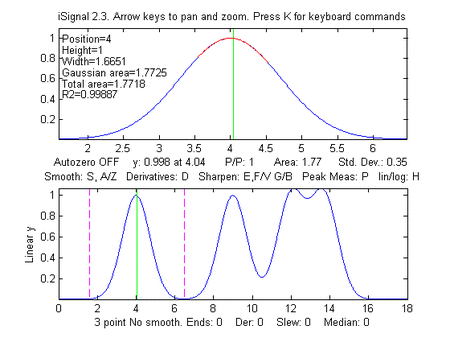>> x=[0:.005:2];y=humps(x);Data=[x;y];

4th derivative of the peak at x=0.9:
>> isignal(Data,0.9,0.5,1,3,1,4); (shown on the right ==>)

Peak sharpening applied to the peak at x=0.3:
>> isignal(Data,0.3,0.5,1,3,1,0,1,220,5400);
(Press 'E' key to toggle sharpening ON/OFF to compare)

EXAMPLE 7: Measurement of peak area.  This example generates four Gaussian peaks, all with the exact same peak height (1.00) and area (1.77). The first peak (at x=4) is isolated, the second peak (x=9) is slightly overlapped with the third one, and the last two peaks (at x= 13 and 15) are strongly overlapped.  To measure the area under a peak using the perpendicular drop method, position the dotted red marker lines at the minimum between the overlapped peaks.

>> x=[0:.01:20];
>> y=exp(-(x-4).^2)+exp(-(x-9).^2)+exp(-(x-13).^2)+exp(-(x-15).^2);
>> isignal(x,y);
`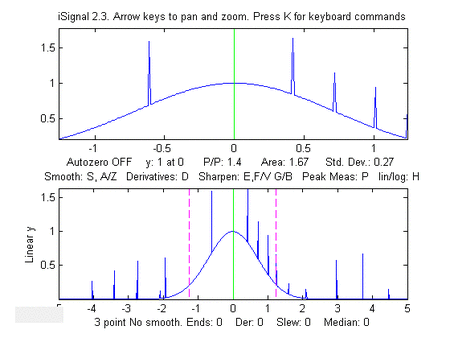`

Greater accuracy in peak area measurement using iSignal can be obtained by using the peak sharpening function to reduce the overlap between the peaks. This reduces the peak widths, increases the peak heights, but has no effect on the peak areas.

EXAMPLE 8: Single peak with random spikes (shown in the figure on the right).
Compare smoothing vs spike filter (
M key) and slew rate limit (~ key) to remove spikes.

`x=-5:.01:5;y=exp(-(x).^2);for n=1:1000, if randn()>2,y(n)=rand()+y(n), end,end;isignal(x,y);`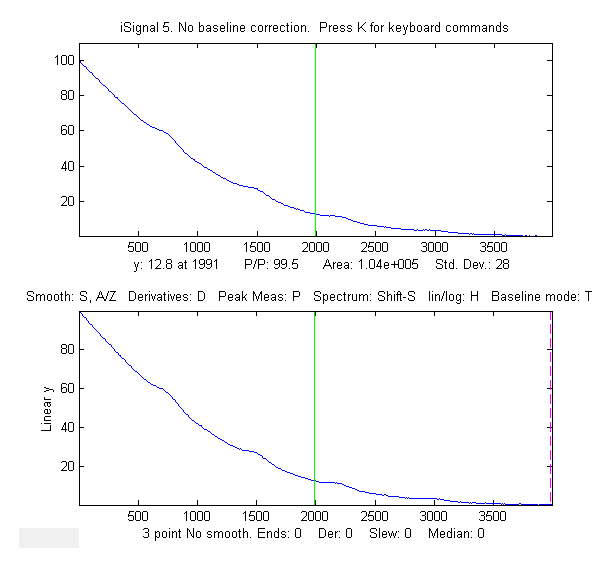EXAMPLE 9: Weak peaks on a strong baseline.
The demo script isignaldemo2 (shown on the left) creates a test signal containing four peaks with heights 4, 3, 2, 1, with equal widths, superimposed on a very strong curved baseline, plus added random white noise. The objective is to extract a measure that is proportional to the peak height but independent of the baseline strength. Suggested approaches: (a) Use automatic or manual baseline subtraction to remove the baseline, measure peaks with the P-P measure in the upper panel; or (b) use differentiation (with smoothing) to suppress the baseline; or (c) use curve fitting (Shift-F), with baseline correction (T), to measure peak height. After running the script, you can press Enter to have the script perform an automatic 3rd derivative calibration, performed by lines 56 to 74. As indicated in the script, you can change several of the constants; search for the word "change". (To use the derivative method, the width of the peaks must all be equal and stable, but the peak positions may vary within limits, set by the Xrange for each peak in lines 61-67). You must have isignal.m and plotit.m installed.

EXAMPLE 10:   Direct entry into frequency spectrum mode, plotting returned frequency spectrum.
>> x=0:.1:60; y=sin(x)+sin(10.*x);
>> [pY,SpectrumOut]=isignal([x;y],30,30,4,3,1,0,0,1,0,0,0,1);
>> plot(SpectrumOut)

EXAMPLE 11:   The demo script demoisignal.m is a self-running demo that requires iSignal 4.2 or later and the latest version of plotit.m to be installed.

EXAMPLE 12:   Here's a simple example of a very noisy signal with lots of high-frequency (blue) noise obscuring a perfectly good peak in the center at x=150, height=1e-4; SNR=90.  First, download NoisySignal into the Matlab path, then execute these statements:

>> isignal(x,y);

Use the A and Z keys to increase and decrease the smooth width, and the S key to cycle through the available smooth type. Hint: use the Gaussian smooth and keep increasing the smooth width. Zoom in on the peak in the center, press P to enter the peak mode, and it will display the characteristics of the peak in the upper left.

KEYBOARD CONTROLS (Version 8):
Pan left and right..........Coarse pan: < and >
Fine pan: left and right cursor arrows
Nudge: [ and ]
Zoom in and out.............Coarse zoom: / and "

Fine zoom: up and down cursor arrows
Resets pan and zoom.........ESC

Select entire signal........Ctrl-A

Display Grid (on/off).......Shift-G  Temporarily displays grid on
the plots
Set smooth width vector.....Shift-Q  for segmented smooth
Adjust smooth type..........S (cycles through None, Rectangular,

Triangle, Gaussian,and Savitzky-Golay)
Toggle smooth ends..........X (0=ends zeroed  1=ends smoothed (slower)
Symmetrize mode             Shift-Y toggles off and on
Shift-1,Shift-2: decrease,increase by 1%
Shift-3,Shift-4: decrease,increase by 1%
order
Toggle peak sharpening......E (0=OFF 1=ON)
Sharpening for Gaussian.....Y  Set sharpen settings for Gaussian
Sharpening for Lorentzian...U  Set sharpen settings for Lorentzian
Adjust sharp2   ............G,B  G=>sharper, B=>less sharpening
Slew rate limit (0=OFF).....~ Largest allowed y change between points
Spike filter width (0=OFF)..M
median filter eliminates sharp spikes
Toggle peak parabola........P  fits parabola to center, labels vertex
Fits peak in upper window...Shift-F (Asks for shape, number of peaks,
Number of trials, etc)
Fit polynomial..............Shift-o  Fits polynomial to data in
upper panel
Find peaks in lower panel...J (Asks for Peak Density)
Find peaks in upper panel...Shift-J (Asks for Peak Density)
Spectrum mode on/off........Shift-S (Shift-A and Shift-X to change
axes)
Peak labels on spectrum.....Shift-Z in spectrum mode
Display Waterfall spectrum..Shift-W  Allows choice of mesh, surf,
contour, or pcolor
Transfer power spectrum.....Shift-T  Replaces signal with power
spectrum
Click graph to print x,y....Shift-C  Click graph to print coordinates
Lock in current processing..Shift-L  Replace signal with processed
version
Power transform method......^ (Shift-6) Raises the signal to the

specified power.
Print peak report...........R  prints position, height, width, area
Toggle overlay mode.........L Overlays original signal as dotted line
Display current signals.....Shift-B  Original (top) vs  Processed
(bottom)
Toggle log y mode...........H  semilog plot in lower window
Cycle baseline mode.........T  none, linear, quadratic, or flat
baseline mode
Restores original signal....Tab key resets to original signal
and modes
Baseline subtraction........Backspace, then click baseline at
multiple points
Restore background..........\  to cancel previous background
subtraction
Invert signal...............-  Invert (negate) the signal (flip + / -)
Remove offset...............0  (zero) set minimum signal to zero
Sets region to zero.........;  sets selected region to zero.
Absolute value..............+  Computes absolute value of entire
signal
Condense signal.............C  Condense oversampled signal by
factor N
Interpolate signal..........i  Interpolate (re-sample) to N points
Print keyboard commands.....K  prints this list
Print signal report.........Q  prints signal info and current
settings
Print isignal arguments.....W  prints isignal (current arguments)
Save output to disk.........O as .mat file with processed signal
matrix
and (in spectrum mode) the
frequency spectrum.

Play signal as sound........Spacebar or Shift-P  Play upper panel

segment through computer sound system
Set sound sample rate.......Shift-R for the Shift-P command.
Switch to ipf.m.............
Shift-Ctrl-F  Transfer current signal to
Interactive Peak Fitter

Switch to iPeak.............Shift-Ctrl-P  Transfer current signal to
Interactive Peak Detector

ProcessSignal, a Matlab/Octave command-line function that performs smoothing and differentiation on the time-series data set x,y (column or row vectors). Type "help ProcessSignal". Returns the processed signal as a vector that has the same shape as x, regardless of the shape of y. The syntax is Processed=ProcessSignal(x, y, DerivativeMode, w, type, ends, Sharpen, factor1, factor2, SlewRate, MedianWidth)

December, 2021. This page is part of "A Pragmatic Introduction to Signal Processing", created and maintained by Prof. Tom O'Haver , Department of Chemistry and Biochemistry, The University of Maryland at College Park. Comments, suggestions and questions should be directed to Prof. O'Haver at toh@umd.edu.